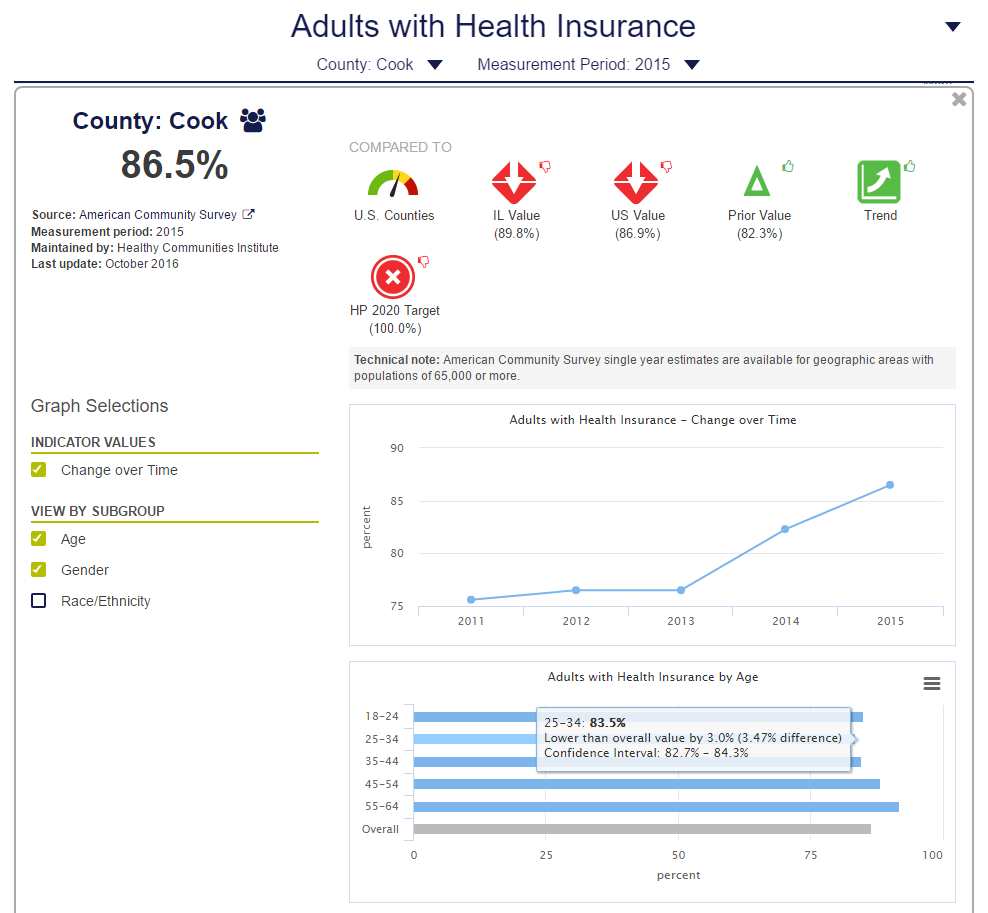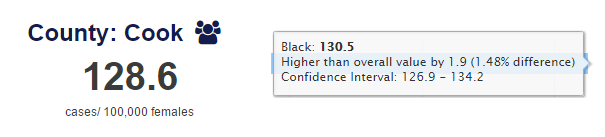# What is the hover-over on subgroup or breakout data?

Many indicators will have subgroup (or breakout) data. A tool-tip appears when you hover-over one of the bars in the chart with information about the breakout value.

1) The first line shows the value of the specific breakout.

• The units for this value will match the main value's units (e.g. %, deaths per 100,000 population, births, etc.)

2) The second line shows by how much the breakout value differs from the main value.

• The units for this value will match the main value's units.
• The values in parenthesis shows the % of difference between the breakout value and the main value. This unit will always be a %. The calculations are as follow: (main value - breakout value)/(main value)*100

3) The third line shows the confidence interval for the value of the breakout.

• The units for this value will be the same as the main value's units.

See the example below where the units are %• The value for adults with health insurance for ages 25-34 is 83.5%.
• The value is lower than the main value (86.5%) by 3.0%. This is a difference of 3.47%; the calculations are as follow: 3.47% = (86.5-83.5)/(86.5)*100.
• The confidence interval for the value of 83.5% is 82.7%-84.3%.

This is another example where the units are not %.

Indicator: Breast Cancer Incidence Rate• The value for breast cancer incidence rate for black women is 130.5 cases per 100,000 females.
• This is higher than the overall value of 128.6 by 1.9 cases per 100,000 females. This is a difference of 1.48%; the calculations are as follow: 1.48% = (128.6-130.5)/(128.6)*100.
• The confidence interval for the value is 126.9-134.2 cases per 100,000 females.